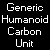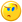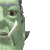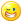## Welcome to the Second Life Forums Archive

These forums are CLOSED. Please visit the new forums HERE

# UTC Date-time vs. UNIX time_t

AnneDroid Lily
Scary robot girl. Rarr.Join date: 10 Nov 2003
Posts: 41
08-02-2005 01:14
The time associated with the email event is given in the UNIX time_t format (as a string). It is the only function or event that uses this format.

Recently, I have become interested in comparing the email time with the UTC Date-time (YYYY-MM-DDTHH:MMS.FFZ) from llGetTimestamp.

Here is what I've come up with for conersions between the two:

From UTC to time_t
CODE
`list julian = [0, 31, 60, 91, 121, 152, 182, 213, 244, 274, 305, 335];integer UTC2time_t(string UTC){    time = llParseString2List(UTC, ["-","T",":","Z"], []);    integer year = llList2Integer(time, 0);    integer month = llList2Integer(time, 1);    integer day = llList2Integer(time, 2);    integer hour = llList2Integer(time, 3);    integer min = llList2Integer(time, 4);    integer sec = llFloor(llList2Float(time, 5) + 0.5);    integer leapDays = 7 + llFloor((year - 2004) / 4);    // my count says that there has been 9 leap days since 1970, but 7 makes the function match an actual timestamp.    seconds = (year - 1970) * 31536000 + (llList2Integer(julian, month - 1) + day + leapDays) * 86400 + hour * 3600 + min * 60 + sec;    return seconds;}`

From time_t to UTC (there is likely a better way to write this)
CODE
`string month;integer date;string time_t2UTC(integer seconds){    string UTC = "";    integer years = llFloor( seconds / 31536000);    integer leapDays = 7 + llFloor((years - 34) / 4);    // my count says that there has been 9 leap days since 1970, but 7 makes the function match an actual timestamp.    seconds = seconds - years * 31536000 - leapDays * 86400;    // seconds will be negative on Jan 1 of a leap year    UTC = (string)(1970 + years);    integer days = llFloor(seconds / 86400);    if (days > 335)    {        month = "-12-";        date = days - 335;    }    else if (days > 305)    {        month = "-11-";        date = days - 305;    }    else if (days > 274)    {        month = "-10-";        date = days - 274;    }    else if (days > 244)    {        month = "-09-";        date = days - 244;    }    else if (days > 213)    {        month = "-08-";        date = days - 213;    }    else if (days > 182)    {        month = "-07-";        date = days - 182;    }    else if (days > 152)    {        month = "-06-";        date = days - 152;    }    else if (days > 121)    {        month = "-05-";        date = days - 121;    }    else if (days > 91)    {        month = "-04-";        date = days - 91;    }    else if (days > 60)    {        month = "-03-";        date = days - 60;    }    else if (days > 31)    {        month = "-02-";        date = days - 31;    }    else    {        month = "-01-";        date = days;    }    if (llFloor((years - 34)/4) == (years - 34) / 4)    {        // if leap year and before feb 29, add the leap day back in.        if (days < 59)        {            date = date + 1;            seconds = seconds + 86400;            if (days == 31)            {                month = "-02-";                date = 1;            }        }        else if (days == 59)        {            month = "-02-";            date = 29;            seconds = seconds + 86400;        }    }    UTC = UTC + month + llGetSubString("0" + (string)date, -2, -1) + "T";    seconds = seconds - days * 86400;    integer hours = llFloor(seconds / 3600);    integer minutes = llFloor((seconds - hours * 3600) / 60);    seconds = seconds - hours * 3600 - minutes * 60;    UTC = UTC + llGetSubString("0" + (string)hours, -2, -1) + ":" + llGetSubString("0" + (string)minutes, -2, -1) + ":" + llGetSubString("0" + (string)seconds, -2, -1) + ".0Z";    return UTC;}`

I hope you find these functions at least moderately useful.
Nada Epoch
The LibrarianJoin date: 4 Nov 2002
Posts: 1,423
Discussion Thread
08-03-2005 09:43
/54/ed/56338/1.html
_____________________
i've got nothing.